Ex 9.6

Chapter 9 Class 12 Differential Equations (Term 2)
Serial order wise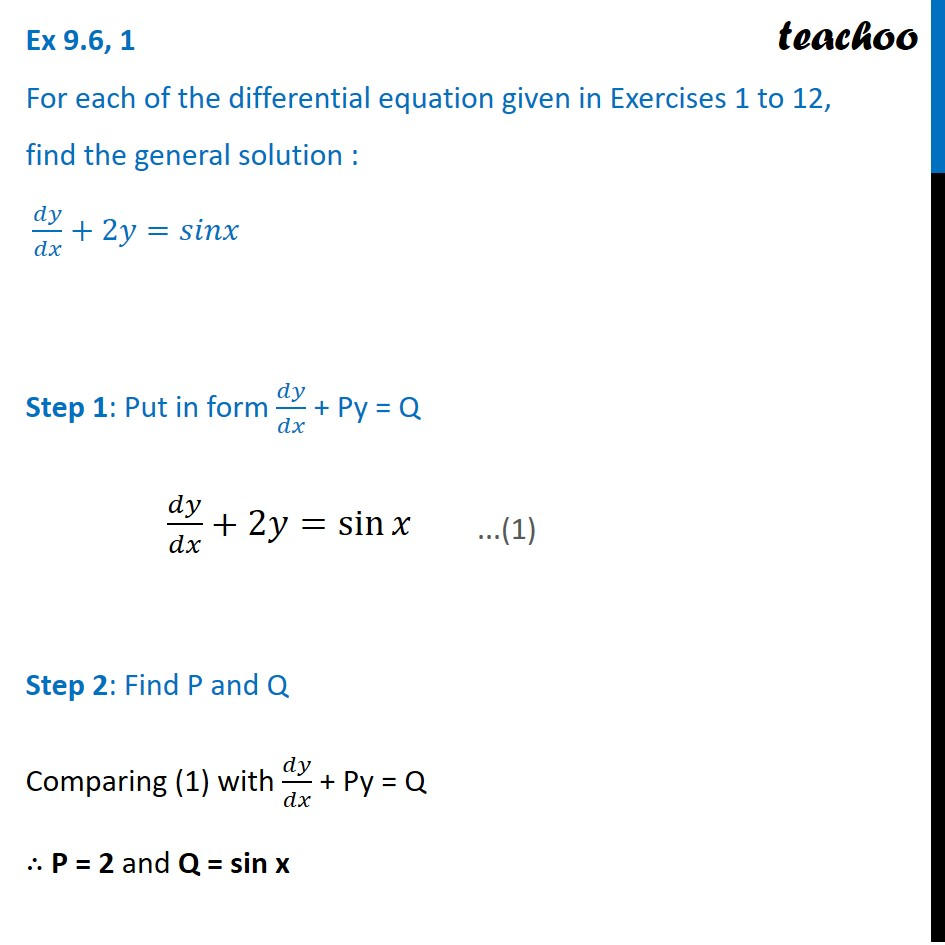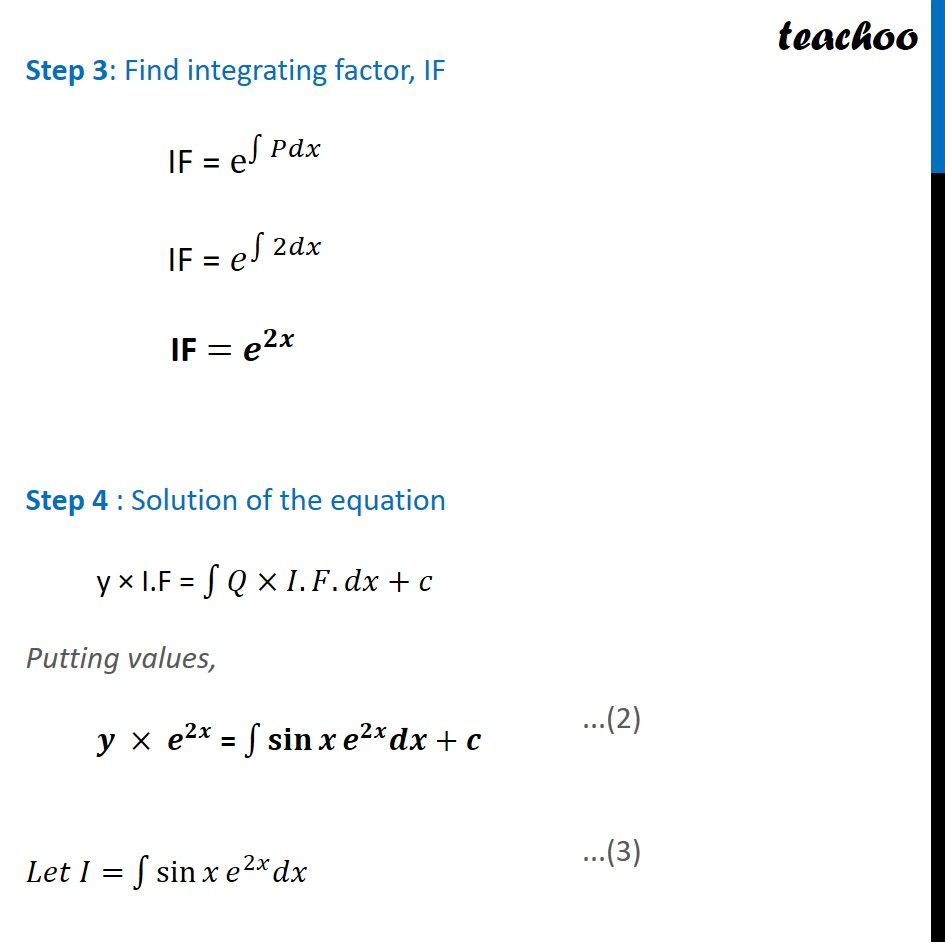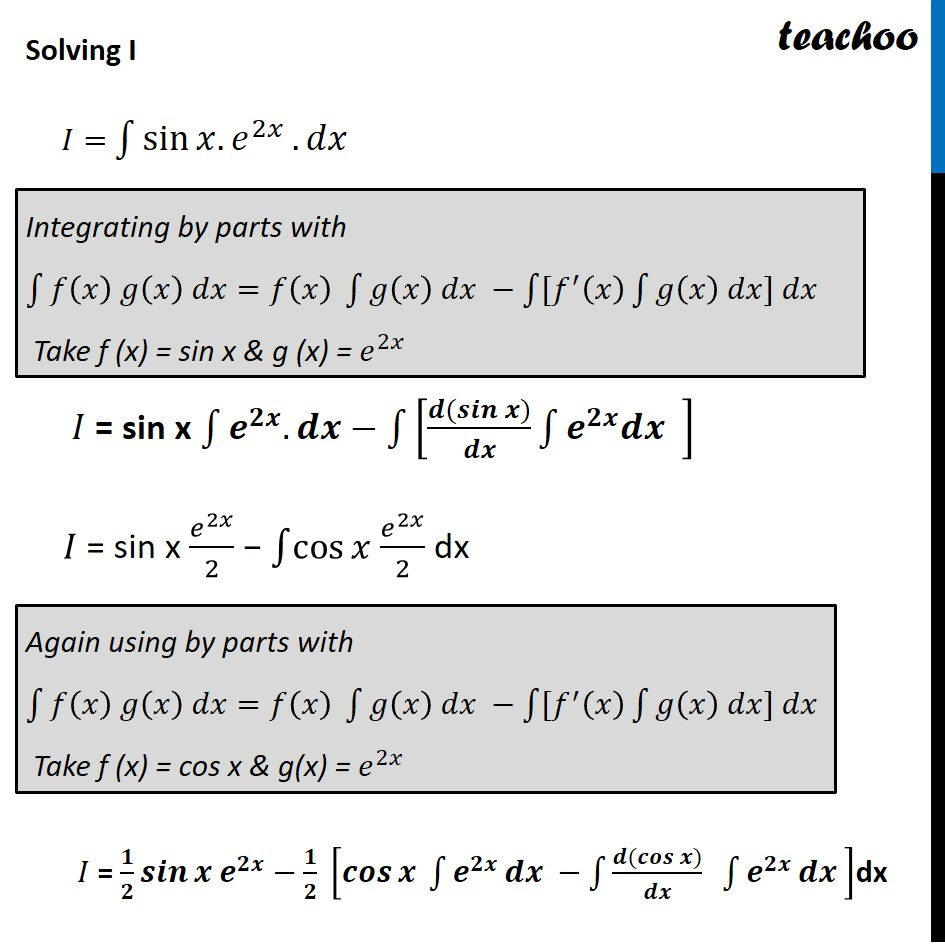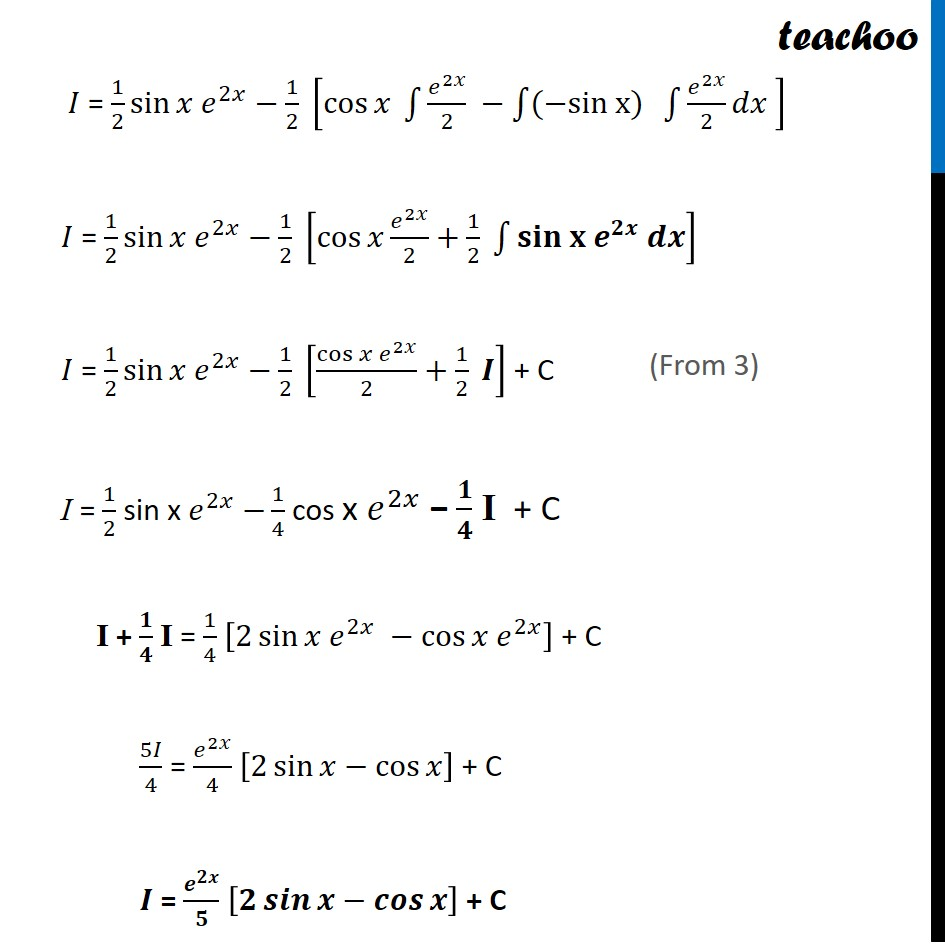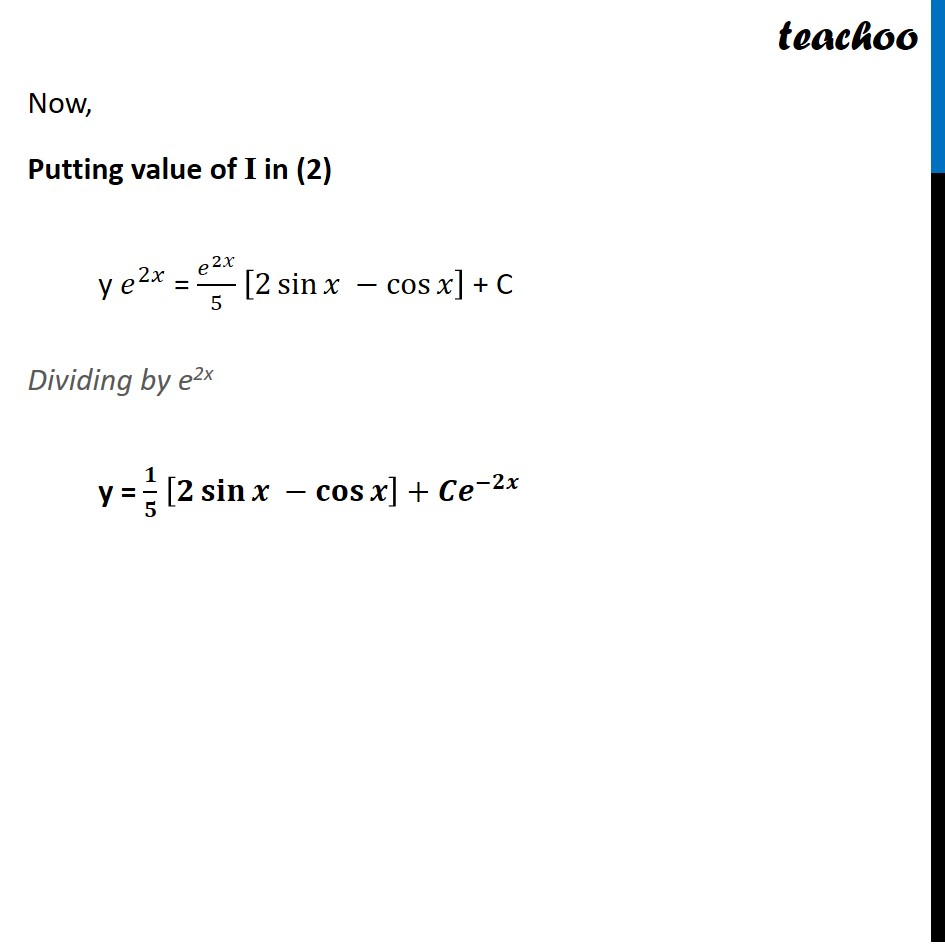### Transcript

Ex 9.6, 1 For each of the differential equation given in Exercises 1 to 12, find the general solution : 𝑑𝑦/𝑑𝑥+2𝑦=𝑠𝑖𝑛𝑥 Step 1: Put in form 𝑑𝑦/𝑑𝑥 + Py = Q 𝑑𝑦/𝑑𝑥+2𝑦=sin⁡𝑥 Step 2: Find P and Q Comparing (1) with 𝑑𝑦/𝑑𝑥 + Py = Q ∴ P = 2 and Q = sin x Step 3: Find integrating factor, IF IF = e^∫1▒𝑃𝑑𝑥 IF = 𝑒^∫1▒2𝑑𝑥 IF = 𝒆^𝟐𝒙 Step 4 : Solution of the equation y × I.F = ∫1▒〖𝑄×𝐼.𝐹.𝑑𝑥+𝑐 〗 Putting values, 𝒚 × 𝒆^𝟐𝒙 = ∫1▒〖𝐬𝐢𝐧⁡𝒙 𝒆^𝟐𝒙 𝒅𝒙〗+𝒄 𝐿𝑒𝑡 𝐼= ∫1▒〖sin⁡𝑥 𝑒^2𝑥 𝑑𝑥〗 Solving I 𝐼= ∫1▒〖sin⁡〖𝑥.𝑒^2𝑥 〗.𝑑𝑥 〗 𝐼 = sin x ∫1▒〖𝒆^𝟐𝒙.𝒅𝒙−∫1▒〖[(𝒅(𝒔𝒊𝒏⁡𝒙))/𝒅𝒙 ∫1▒〖𝒆^𝟐𝒙 𝒅𝒙 〗] 〗 〗 𝐼 = sin x 𝑒^2𝑥/2 − ∫1▒cos⁡𝑥 𝑒^2𝑥/2 dx 𝐼 = 𝟏/𝟐 𝒔𝒊𝒏⁡〖𝒙 𝒆^𝟐𝒙 〗−𝟏/𝟐 [𝒄𝒐𝒔⁡𝒙 ∫1▒𝒆^𝟐𝒙 𝒅𝒙 −∫1▒(𝒅(𝒄𝒐𝒔⁡𝒙))/𝒅𝒙 ∫1▒𝒆^𝟐𝒙 𝒅𝒙 ]dx Integrating by parts with ∫1▒〖𝑓(𝑥) 𝑔(𝑥) 𝑑𝑥=𝑓(𝑥) ∫1▒〖𝑔(𝑥) 𝑑𝑥 −∫1▒〖[𝑓^′ (𝑥) ∫1▒〖𝑔(𝑥) 𝑑𝑥] 𝑑𝑥〗〗〗〗 Take f (x) = sin x & g (x) = 𝑒^2𝑥 Again using by parts with ∫1▒〖𝑓(𝑥) 𝑔(𝑥) 𝑑𝑥=𝑓(𝑥) ∫1▒〖𝑔(𝑥) 𝑑𝑥 −∫1▒〖[𝑓^′ (𝑥) ∫1▒〖𝑔(𝑥) 𝑑𝑥] 𝑑𝑥〗〗〗〗 Take f (x) = cos x & g(x) = 𝑒^2𝑥 𝐼 = 1/2 sin⁡〖𝑥 𝑒^2𝑥 〗−1/2 [cos⁡𝑥 ∫1▒𝑒^2𝑥/2 −∫1▒〖(−sin x)〗 ∫1▒𝑒^2𝑥/2 𝑑𝑥 ] 𝐼 = 1/2 sin⁡〖𝑥 𝑒^2𝑥 〗−1/2 [cos⁡𝑥 𝑒^2𝑥/2+1/2 ∫1▒〖𝐬𝐢𝐧 𝐱 𝒆^𝟐𝒙 𝒅𝒙〗] 𝐼 = 1/2 sin⁡〖𝑥 𝑒^2𝑥 〗−1/2 [(cos⁡𝑥 𝑒^2𝑥)/2+1/2 𝑰] + C I = 1/2 sin x 𝑒^2𝑥 −1/4 cos x 𝑒^2𝑥 − 𝟏/𝟒 I + C I + 𝟏/𝟒 I = 1/4 [2 sin⁡〖𝑥 𝑒^2𝑥 −cos⁡〖𝑥 𝑒^2𝑥 〗 〗 ] + C 5𝐼/4 = 𝑒^2𝑥/4 [2 sin⁡〖𝑥−cos⁡𝑥 〗 ] + C 𝑰 = 𝒆^𝟐𝒙/𝟓 [𝟐 𝒔𝒊𝒏⁡〖𝒙−𝒄𝒐𝒔⁡𝒙 〗 ] + C Now, Putting value of I in (2) y 𝑒^2𝑥 = 𝑒^2𝑥/5 [2 sin⁡〖𝑥 −cos⁡𝑥 〗 ] + C Dividing by e2x y = 𝟏/𝟓 [𝟐 𝐬𝐢𝐧⁡〖𝒙 −𝐜𝐨𝐬⁡𝒙 〗 ]+𝑪𝒆^(−𝟐𝒙)